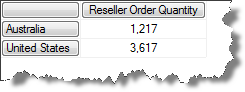## MDX equivalent of a filtered GROUP BY in SQL

Does that title make sense? I don't know if it does, but I can't think of another description for this problem. If anyone can think of a better title I would love to hear it. It's hard to explain in words so let's jump into some code examples.

Consider the following SQL statement against the AdventureWorksDW relational database. The requirement is to select a list of 4 cities and then want to see the order quantity grouped at the country level.

```SELECT
g.EnglishCountryRegionName Country
,sum(OrderQuantity) as OrderQuantity
FROM dbo.FactResellerSales rs
INNER JOIN dimReseller r
ON r.ResellerKey = rs.ResellerKey
INNER JOIN dimGeography g
ON g.GeographyKey = r.GeographyKey
WHERE g.City IN ('Melbourne','Sydney','Seattle','New York')
GROUP BY g.EnglishCountryRegionName
```

This query returns the following result: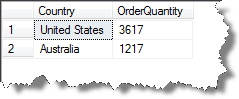How can we do an equivalent query in MDX?

if you start with something like the following it gets you the correct raw figures, but it breaks the amounts out for each city and we want to see the sub-totals by country.

```SELECT
[Measures].[Reseller Order Quantity] on 0
,{[Geography].[City].[Melbourne]
,[Geography].[City].[Sydney]
,[Geography].[City].[Seattle]
,[Geography].[City].[New York]} on 1
```

This is the result that you get: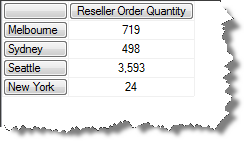So then you might figure that putting the country members on the axis and the cities in the where clause should do the trick.

```SELECT
{Measures.[Reseller Order Quantity]} on 0
,[Geography].[Country].[Country].Members on 1
WHERE (
{[Geography].[City].[Melbourne]
,[Geography].[City].[Sydney]
,[Geography].[City].[Seattle]
,[Geography].[City].[New York]})
```

But then we get the following...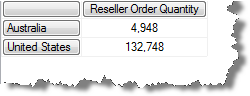So what is going on here? The query has returned the total amounts for the entire countries, not just the sub totals for the cities in the WHERE clause. Because of the attribute relationship between Countries and Cities, SSAS has put the country members on the rows that are related to the cities in the WHERE clause. In effect what this query is roughly saying to SSAS is "show me the Reseller Order Quantity for the Countries that contain one or more of the following cities. There is a more in depth explanation of this behavior here: Attribute Relationships Explained

So what we want to do is to get a measure that is filtered to figures for the selected cities. One way of doing this would be to created a calculated measure like the following:

```WITH MEMBER Measures.FilteredSales as
SUM(
EXISTING {[Geography].[City].[Melbourne]
,[Geography].[City].[Sydney]
,[Geography].[City].[Seattle]
,[Geography].[City].[New York]}
, [Measures].[Reseller Order Quantity])
SELECT
{Measures.[FilteredSales]} on 0
,[Geography].[Country].[Country].Members on 1
WHERE (
{[Geography].[City].[Melbourne]
,[Geography].[City].[Sydney]
,[Geography].[City].[Seattle]
,[Geography].[City].[New York]})```

This gets the result that we are after, but it was a rather convoluted solution.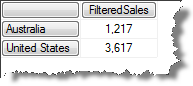However, there is an easier way. We could use a sub-select...

```SELECT
[Measures].[Reseller Order Quantity] on 0,
[Geography].[Country].[Country].Members on 1
FROM (    SELECT
{[Geography].[City].[Melbourne]
,[Geography].[City].[Sydney]
,[Geography].[City].[Seattle]
,[Geography].[City].[New York]} on 0